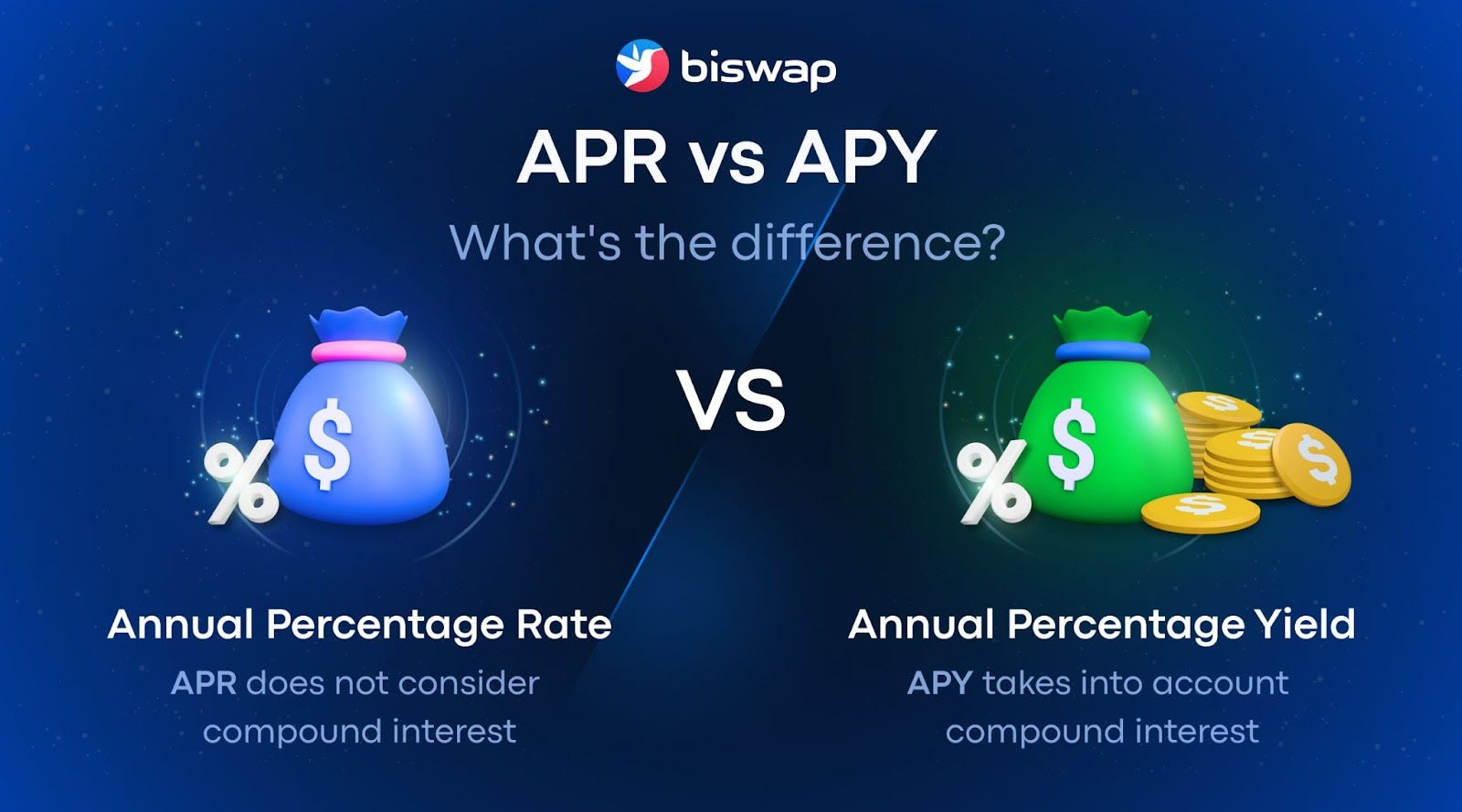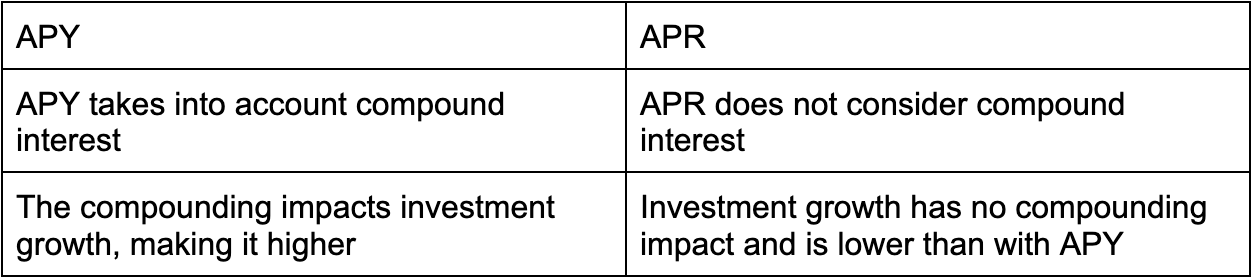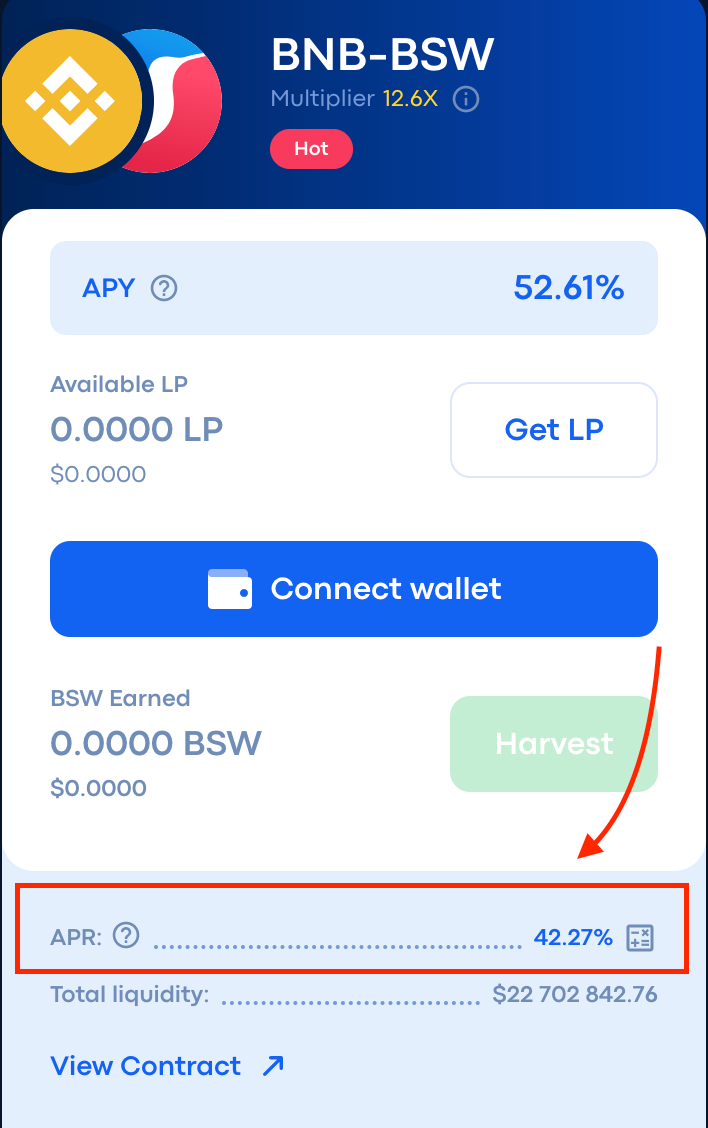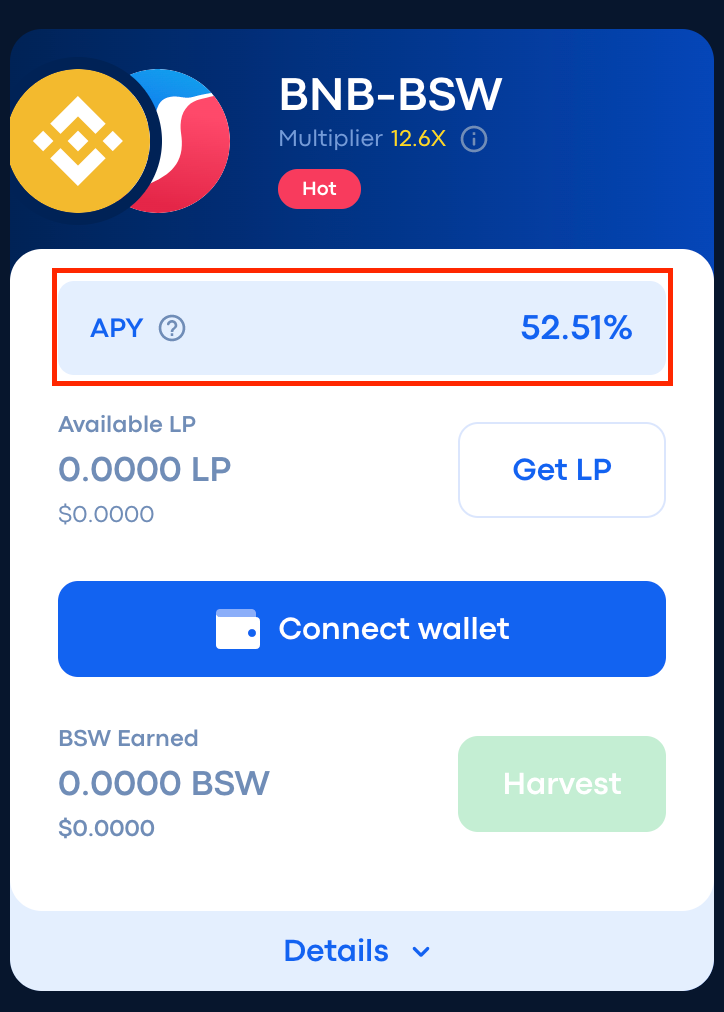Comment on page

# APR vs APY Overview

What’s the difference?APY and APR in crypto are used to calculate interest on investment, and both significantly affect how much you will earn saving crypto assets or lending crypto assets.
• APY (Annual Percentage Yield)
• APR (Annual Percentage Rate)
Here is a short overview of the difference and how to calculate your investment growth:With compound interest, the amount of return that investors get is higher because the interest is calculated from the principal + the previously earned interest. So, in addition to the interest paid on the principal amount, the reward will continue to increase due to the interest that has accumulated during the time.

### How does it work on Biswap?

Let’s take as an example investing in Biswap Farms:
APR on Biswap represents the annual percentage income for staking tokens
In case you do Manual Compound, that will make your profit higher since the rewards earned are added to the initial deposit and start generating income as well.
For Example:APR is calculated by summing up the rewards of the liquidity providers and the rewards in BSW
You approved 1 000 BSW on a farm under 40% APR. That means that at the time of calculation, your income for 1 year can be calculated as follows:
1 000 BSW (the invested sum) x 40 % (APR) / 100 = 400 BSW — your 1-year income.
To calculate the daily income, you need:
1. 1.
40% (APR) / 365 (days of the year) ~ 0.001% (your daily income in percentage)
2. 2.
1 000 BSW (the invested sum) x 0.001% of your daily income in percentage) / 100 (also can be counted as 1 000 x 0.001 %) ~ 1 BSW — your daily income in BSW
That means that your 1-year total sum potentially can be:
1 000 BSW (if you unstake your deposit sum) + 400 BSW (your income  —  the sum of APR) ~ 1 400 BSW
Keep in mind that the rates are approximate, provided for your convenience only, and in no way represent a guaranteed return. All the mathematical calculations aren’t financial advice.
APY (Annual Percentage Yield) on Biswap   is the annual percentage of return earned from staking, taking into account the automatic increase of the invested sum.
For Example:APY is based on your one-year income if Harvest and Compound are made once a day. Provided APY calculations depend on current APR rates.
You approved 1 000 BSW in BSW farms for Auto-compounding under 60% APY.
That means that at the time of calculation, your income for the 1 year can be calculated:
1 000 (the invested sum) x 60% (APY in percentage) / 100 (also can be counted as 1 000 x 60% )= 600 BSW — your 1- year income if you won’t click harvest during this year.
Pay attention that you cannot accurately calculate your daily income because of permanent automatic change and an increase in your investment sum.
That means that your 1-year total sum potentially can be:
1 000 BSW (if you harvest your deposit sum) + 6 00 BSW (your income — the sum of APY) = 1 600 BSW
Keep in mind that the rates are approximate, provided for your convenience only, and in no way represent a guaranteed return. All the mathematical calculations aren’t financial advice.

### Why do the APY & APR percentages change?

The APR & APY percentages change dynamic depending on the number of funds locked in the certain Biswap Launchpool or Farm.
There is a principle of its changes:
The more users stake their tokens in Launchpools or Farms, the lower APR & APY percentages will be.
It means that APR & APY percentages will change in case of the high demand on a certain Launchpool or Farm.